# Python decimal - division, round, precision

Published on August 3, 2022By ShubhamWhile we believe that this content benefits our community, we have not yet thoroughly reviewed it. If you have any suggestions for improvements, please let us know by clicking the “report an issue“ button at the bottom of the tutorial.

Python decimal module helps us in division with proper precision and rounding of numbers.

## Python decimal module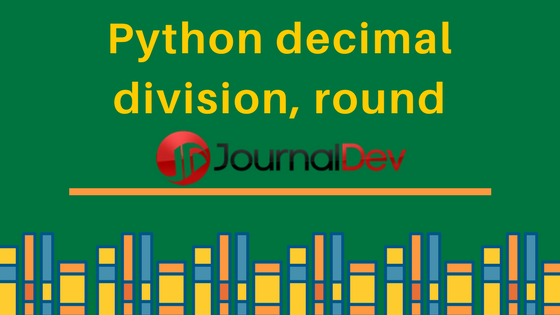In this lesson on decimal module in Python, we will see how we can manage decimal numbers in our programs for precision and formatting and making calculations as well. The precision with decimal numbers is very easy to lose if numbers are not handled correctly. Let’s see how the decimal module and its available functions help in these areas.

## Working with decimal numbers

Decimal numbers are just the floating-point numbers with fixed decimal points. We must round off the numbers correctly based on our need, otherwise, the results can be unexpected. Python’s decimal module helps us to be more precise with decimal numbers.

## Need for decimal module

Before actually putting this module to use, let’s see what precision are we talking about and establish why we need this module actually. Look at the following code snippet:

``````division = 72 / 7
print(division)
``````

Let’s see the output for this program: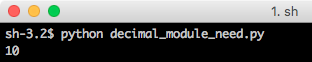Well, the answer was not actually accurate and there were no decimal points at all! Let’s see how we can correct this by using the decimal module.

## Using the decimal module

In all the programs we make in this post, we will be importing the decimal module in all of them:

``````import decimal
``````

It might be the case that we only import a specific function from this module. This can be done as:

``````from decimal import Decimal
``````

Let’s start putting the decimal module into some programs.

## Python decimal module example

### Correcting Division with decimals

Here, we will correct the program we wrote above to perform division which should have produced a floating-point result. Modified program with the decimal module will look like:

``````import decimal

division = decimal.Decimal(72) / decimal.Decimal(7)
print(division)
``````

Let’s see the output for this program: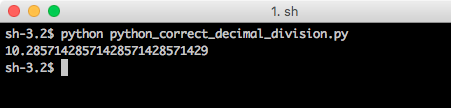To notice, the division is correct now and precise as well, or maybe it is too precise?

### Controllling Precision for single operation

In the last program, there were 25 decimal places in the answer now. But what if we only wanted up to three places of decimal values? This can be controlled as well. Here, we will control the precision of the answer which will not reflect in other operations in our program:

``````import decimal

with decimal.localcontext() as ctx:
ctx.prec = 3
division = decimal.Decimal(72) / decimal.Decimal(7)
print(division)

again = decimal.Decimal(72) / decimal.Decimal(7)
print(again)
``````

We did the division operation two times to prove a point. Let’s see the output for this program: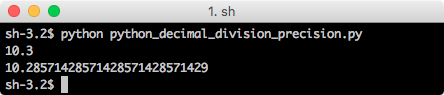Noticed something? The precision we set was only valid for a single time. Next time we did the division, we got back the same result.

### Controllling Precision for complete program

It is also possible to control precision globally in the program. This is not recommended all the time when you are dealing with a lot of numbers in your program. Here is an example:

``````import decimal

decimal.getcontext().prec = 3

division = decimal.Decimal(72) / decimal.Decimal(7)
print(division)

again = decimal.Decimal(72) / decimal.Decimal(7)
print(again)
``````

Let’s see the output for this program: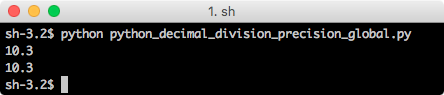### Rounding the numbers

It is possible to gracefully round the numbers with the `round(...)` function. Let’s try it:

``````import decimal

#Can be rounded to 13.48 or 13.49
rounded = round(13.485, 2)
print(rounded)
``````

Let’s see the output for this program: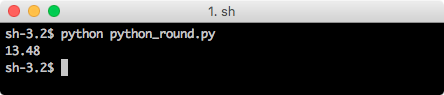The number in program can be rounded to 13.48 or 13.49. By default, the `round(...)` function rounds down. This can be changed as well:

``````import decimal

#Can be rounded to 13.48 or 13.49
rounded = round(13.485, 2)
print(decimal.Decimal(rounded).quantize(decimal.Decimal('0.00'), rounding=decimal.ROUND_UP))
``````

Let’s see the output for this program: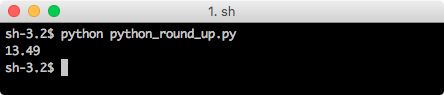## Getting Python Decimal Context

If you are interested looking at default context set by default for decimal module, you can use the following script:

``````from decimal import *
print(getcontext())
``````

Let’s see the output for this program: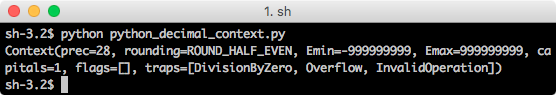That’s all for python decimal module, it’s very useful when working with float point numbers.

Thanks for learning with the DigitalOcean Community. Check out our offerings for compute, storage, networking, and managed databases.Shubham

author

#### Still looking for an answer?

Ask a questionSearch for more help

Click below to sign up and get \$200 of credit to try our products over 60 days!

### Popular Topics

##### Join the Tech Talk##### Hollie's Hub for Good

Working on improving health and education, reducing inequality, and spurring economic growth? We’d like to help.